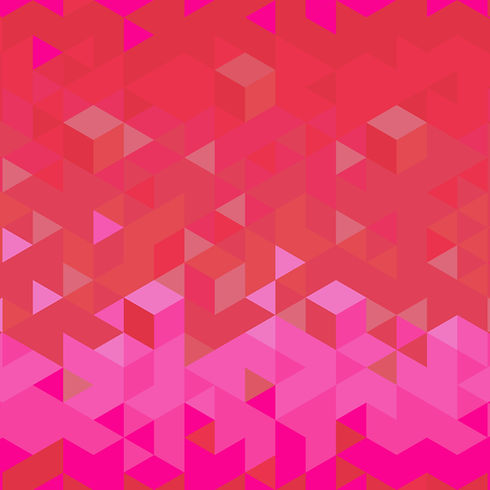top of page
• rishabhdwivedi062

# Types of graphs used in machine learning

Updated: Oct 6, 2022

Graphs are the most useful thing used in data exploration and feature engineering part of machine learning. With the help of graphs, we can find out the patterns and can meaningful insights into data.

#### Let's discuss various kinds of graphs present in ML.

1. Line Graph: Line graph show us the relationship between the two variables. With this graph, we can find our trends at a different points. For example, for a particular value of X we can find the value of Y.2. Bar Graph:

A bar graph contains gaps among different points and hence used to find the value

X with respect to Y.3. Histogram :

A histogram is an approximate representation of the distribution of numerical data.

It does not contain gaps.4. Pie Chart:

A pie chart is a circular statistical graphic, which is divided into slices to illustrate

numerical proportion.5. Scatter Plot:

A scatter plot is a type of mathematical diagram using cartesian coordinates to

display values for typically two variables for a set of data.6. Box and Whisker Plot:

It is the graphical representation of the statical summary of numeric variables. Here

the parts are divided into quadrants and the lower part represents the minimum

value and upper part maximum value.7. Time Series Graph:

Time series is a series of data points indexed in time order. Most commonly, a time

series is a sequence taken at successive equal points in time.That's all, hope you have learned something new, we will discuss this in detail in further blogs.